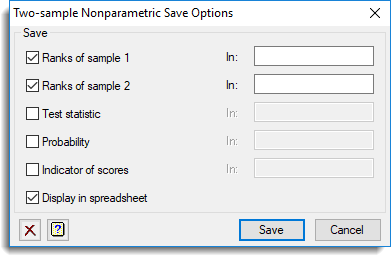1. Home
2. Two-sample Nonparametric Tests Save Options

# Two-sample Nonparametric Tests Save Options

Use this to save results from a two-sample nonparametric test in Genstat data structures.

1. After selecting the appropriate boxes, type the names for the identifiers of the data structures into the corresponding In: fields.## Save

The available save options for each type of test are as follows:

 Mann-Whitney U Test Ranks of sample 1 Variate Saves ranks of sample 1 or both samples if data specified in groups Ranks of sample 2 Variate Saves ranks of sample 2 (when data is supplied in 2 variates) Test statistic Scalar Saves the test statistic U Probability Scalar Saves the Normal approximation to the test statistic Indicator of scores Scalar Saves an indicator: 1 if the first sample scores the highest ranks on average, 0 otherwise Wilcoxon matched-pairs test Ranks Variate Saves signed ranks Statistic Scalar Saves the test statistic Normal approximation Scalar Saves the Normal approximation of test Sign of ranks Scalar Indicates the sign of the total sum of the signed ranks Kolmogorov-Smirnoff test Ranks of sample 1 Variate Saves ranks of sample 1 Ranks of sample 2 Variate Saves ranks of sample 2 Test statistic Scalar Saves the test statistic (the maximum absolute difference between the cumulative distribution functions) Chi-square approximation Scalar Saves the chi-square approximation to the test statistic Signed differences Variate Saves the signed differences between the cumulative distribution functions Sign test Test statistic Scalar Saves the sign test statistic Effective sample size Scalar Saves the effective sample size Probability level Scalar Saves the probability level of test Spearman’s rank correlation Rank correlation coefficient Scalar Saves the rank correlation coefficient Student’s t approximation Scalar Saves the Student’s t approximation to the correlation coefficient Degrees of freedom Scalar Saves the degrees of freedom for each t statistic Ranks Variate Saves the ranks